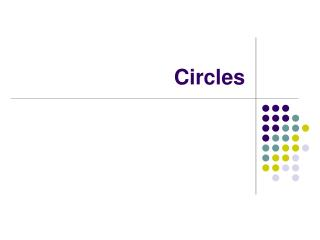# Circles - PowerPoint PPT PresentationDownload PresentationCircles

CirclesDownload Presentation## Circles

- - - - - - - - - - - - - - - - - - - - - - - - - - - E N D - - - - - - - - - - - - - - - - - - - - - - - - - - -
##### Presentation Transcript

1. Circles

2. Central Angles • The vertex of a central angle is at the center of the circle. <AMB is a central angle. • The sides of a central angle are radii of the circle. (AM and MB) • An arc is a portion of the circle. AB is an arc. • The measure of the intercepted arc of a central angle is equal to the measure of the central angle. (Measured in degrees) • A chord is a segment whose endpoints are on the circle. (AB) D V M A B

3. Arcs of Circles • A minor arc measures less than 180°. (Two letters) • A major arc measures more than 180°. (Three letters) • A semicircle is an arc that measures exactly 180° (half a circle) and it is bound by the diameter of a circle. D V M Name the following: A minor arc: ___________ A major arc: ___________ A semicircle: ___________ A B

4. Arcs of Circles Name the following: Two central angles: _____________ Find mDV: ____________________ Find mVB: ____________________ Find mDVB: ___________________ Find mVAB: ___________________ Find m VBD: ___________________ Name one of the longest chords: _________ What fractional part of the circle is AB? _______ D V M A B 64˚

5. Area of Sector • The sector of a circle is the region defined by two radii of a circle. The area of a sector is a portion of the total area of the circle. To find the portion, take the Measure of the arc/360° and multiply by the total area of the circle. D V The area of sector DMV = The area of sector VMB = M 6 in A B 30˚

6. Arc Length • Arc length is a portion of the circumference of a circle. To find the arc Length, take the Measure of the arc/360° and multiply by the total circumference of the circle. D V The length of AB = The length of VB = M 6 in A B 64˚

7. Inscribed Angles • The vertex of an inscribed angle is a point on the circle. • The sides of an inscribed angle are chords of the circle. • The measure of an inscribed angle is half the measure of its intercepted arc. Q 64° P R

8. F D A B C Inscribed angles • Name the inscribed angle for arc AFB • What does this angle measure? • What does AC measure? • What does BC measure? • What does <BAC measure? 41°

9. Inscribed angles • Name the inscribed angle for arc CAF. • What does arc AF measure? • What does BC measure? • What does <ACB measure? • What does <AFB measure? • What does <FAC measure? F 88° D A B 68° 76° C

10. Circle Proportions • In Circle Y with central angle <XYZ, all of the following ratios are equal: 60° x Find the length of XZ: Find the area of sector XYZ: z Y

11. Worksheet • 6.7 Arc Length • 8.6 Sectors

12. B D A C Secants and tangents • A secant line intersects a circle in two points. Line CD is a secant line. • A tangent line intersects a circle in one point. Line AB is a tangent line.

13. B D A A C C B Tangent Properties • If a line is tangent to a circle, then it is perpendicular to the radius at the point of tangency. • Two tangent segments to a circle from the same exterior point are congruent.

14. Chords • The perpendicular bisector of a chord contains the center of the circle. • If a radius of a circle bisects a chord of the circle that is not a diameter, then it is perpendicular to the chord. • If a radius of a circle is perpendicular to a chord of the circle, then it bisects the chord. • In a circle, minor arcs are congruent if and only if they have congruent chords.

15. A C B Tangent-Tangent Angle Theorem • The measure of a tangent-tangent angle is equal to one-half the difference of the measures of its two intercepted arcs. D 130°

16. Secant-Secant Angle Theorem • The measure of a secant-secant angle is equal to one-half the difference of the measures of its two intercepted arcs. E A 30° C 140° B D

17. Tangent-Secant Angle Theorem • The measure of a tangent-secant angle is equal to one-half the difference of the measures of its two intercepted arcs. A 160° 70° C B D

18. Worksheet • 6.3 Arcs and Angles